# Count Specific Characters in Column – Excel & Google Sheets

This tutorial will demonstrate how to count specific characters in a column in Excel and Google Sheets.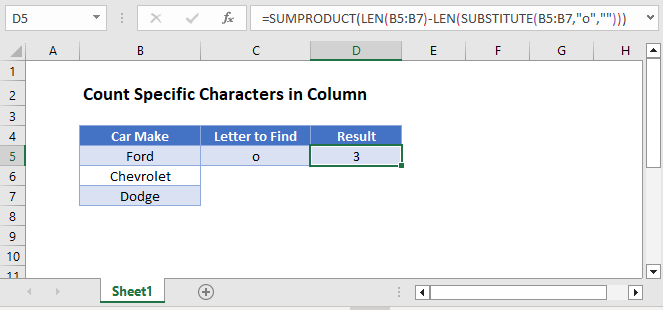## SUMPRODUCT, LEN and SUBSTITUTE Functions

To count the number of specific characters in a column we can use the SUMPRODUCT, LEN and SUBSTITUTE Functions.

``=SUMPRODUCT(LEN(B3:B5)-LEN(SUBSTITUTE(B3:B5,"o","")))``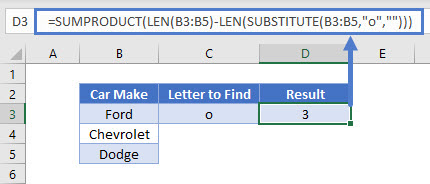### SUBSTITUTE Function

First, we can remove the letter “o” from the column by using the SUBSITUTE Function:

``=SUBSTITUTE(B3:B5, "o", "")``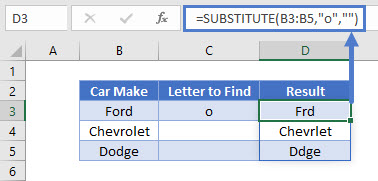This will remove the “o” from all the words in column B.

## LEN Function

Next the LEN function can be used to give us the length of the text in the cell range selected, firstly with the letter ‘o’ and secondly without the letter ‘o’

``=LEN(B3:B3)``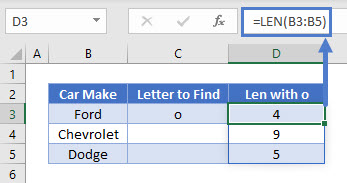``=LEN(SUBSTITUTE(B3:B5, "o", "")``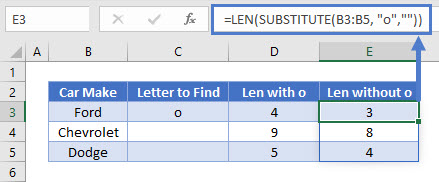If we then subtract the second formula from the first formula we will get the number of the letter “o”‘s in each cell in the range.

``=LEN(B3:B5)-LEN(SUBSTITUTE(B3:B5,"o",""))``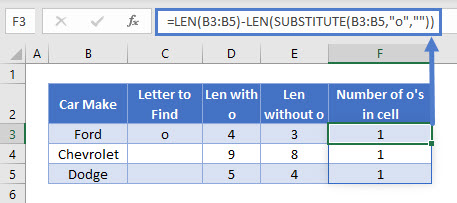## SUMPRODUCT Function

Finally, the SUMPRODUCT Function brings the entire formula to show the number of “o” in the column as a total.## Count Specific Characters in a Column in Google Sheets

The example above works the same way in google sheets.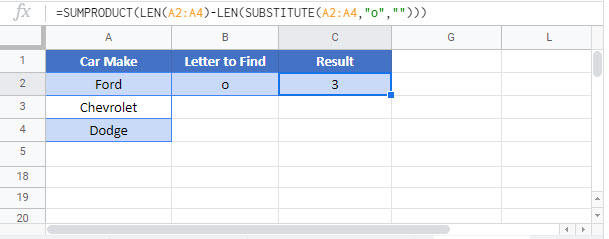### Excel Practice Worksheet

Practice Excel functions and formulas with our 100% free practice worksheets!